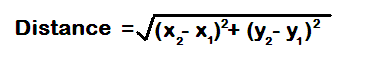# Distance Formula Calculator

Find the distance between two points from x and y coordinates with this distance formula calculator.

Coordinates of Point 1 (x1,y1):
x=
y=

Coordinates of Point 2 (x2,y2):
x=
y=

Calculate the distance between two points by filling in the x and y
values of both coordinates.

## How do you find the distance between two points?

Distance Formula :Example:

For two points, (3,2) and (15, 10) the distance is calculated as:Distance = 14.42 (rounded to the nearest 100th)

## More common examples to try with the Distance Formula Calculator.

What is the distance between points f(2, 9) and g(4, 14)? If you apply the distance formula, or insert it into the calculator, you'd find an answer of 5.39 units. If you round this to the nearest whole number, this would be 5.

What is the distance between points f(6, 4) and g(14, 19)? Using the distance formula calculator, this gives an even result of 17 units.

Try two points with one as a negative, f(2, 9) and g(-2, 6)? Using the distance formula calculator, this gives an even result of 5 units.

What is the distance between points f(3, 12) and g(14, 2)? The answer is 14.87 units.

What is the distance between points f(13, 2) and g(7, 10)? The result is 10 units.

Try these coordinates with some negatives,(-6, -1) and g(4, 3). Using the distance formula calculator, this gives a result of 10.77 units.

What is the distance between points f(-5, -2) and g(2, 4)? Using the distance formula calculator, this gives a result of 9.22 units.

## Euclidean Distance

This distance formula that's used by the calculator is also known as the standard Euclidean distance formula. This concept is named after the Greek mathemetician, Euclid. His writings in Elements were used as a standard textbook for many centuries. Much of what we use in Geometry can be found in his writings. Another concept, the Pythagorean Theorem, is the backbone of this distance formula and how to find the distance between two points on a plane by using the theorem to find a hypotenuse of a triangle.